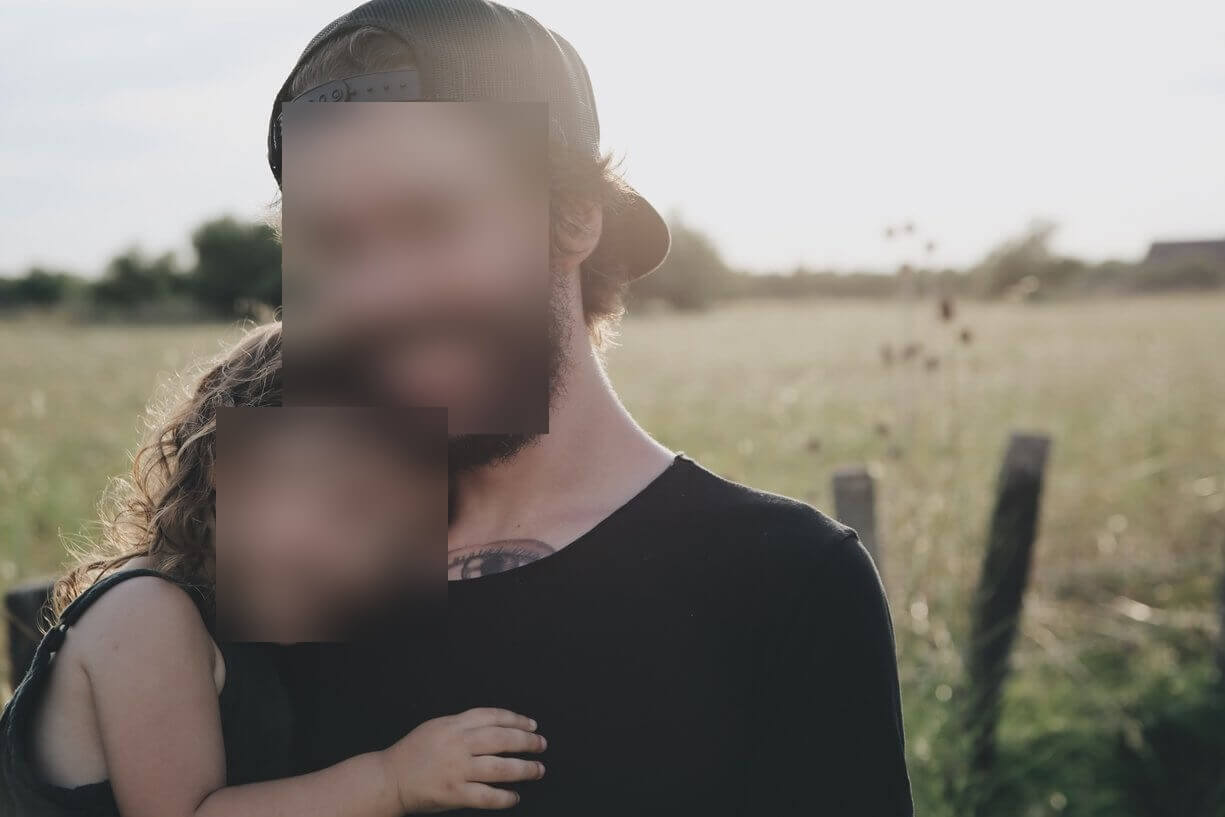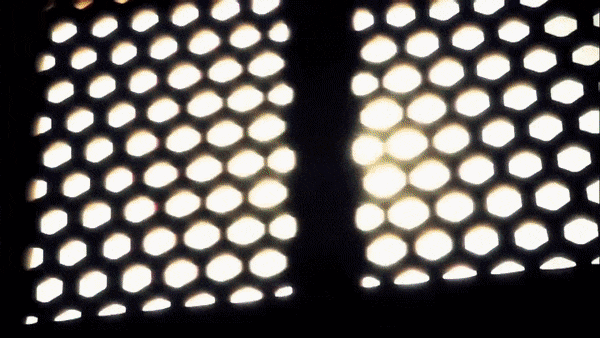# How to Blur Faces in Images using OpenCV in Python

Blurring and anonymizing faces in images and videos after performing face detection using OpenCV library in Python.
4 min read · Updated jul 2022 · Machine Learning · Computer Vision

Disclosure: This post may contain affiliate links, meaning when you click the links and make a purchase, we receive a commission.

In many cases, you want to upload a video or an image publicly on the Internet, and you may want to anonymize random people shown in that video or image. In this tutorial, you will learn how you can blur faces in images and videos using the OpenCV library in Python.

In order to blur faces shown in images, you need to first detect these faces and their position in the image. Luckily for us, I already wrote a tutorial on face detection, we'll only be using its source code, feel free to check it out for further detail on how the face detection code works.

To get started, installing the required dependencies:

``pip3 install opencv-python numpy``

Open up a new file and import:

``````import cv2
import numpy as np``````

As explained in the face detection tutorial, since we need to initialize our deep learning model to detect faces, we need to get the model architecture along with its pre-trained weights, download them, and put them in the weights folder:

``````# https://raw.githubusercontent.com/opencv/opencv/master/samples/dnn/face_detector/deploy.prototxt
prototxt_path = "weights/deploy.prototxt.txt"
# https://raw.githubusercontent.com/opencv/opencv_3rdparty/dnn_samples_face_detector_20180205_fp16/res10_300x300_ssd_iter_140000_fp16.caffemodel
model_path = "weights/res10_300x300_ssd_iter_140000_fp16.caffemodel"

The below code reads this image, prepares it, and pass it to the neural network:

``````# read the desired image
# get width and height of the image
h, w = image.shape[:2]
# gaussian blur kernel size depends on width and height of original image
kernel_width = (w // 7) | 1
kernel_height = (h // 7) | 1
# preprocess the image: resize and performs mean subtraction
blob = cv2.dnn.blobFromImage(image, 1.0, (300, 300), (104.0, 177.0, 123.0))
# set the image into the input of the neural network
model.setInput(blob)
# perform inference and get the result
output = np.squeeze(model.forward())``````

Now, the `output` object is a NumPy array that has all faces detected, let's iterate over this array and only blur portions where we're confident that it's a face:

``````for i in range(0, output.shape):
confidence = output[i, 2]
# get the confidence
# if confidence is above 40%, then blur the bounding box (face)
if confidence > 0.4:
# get the surrounding box cordinates and upscale them to original image
box = output[i, 3:7] * np.array([w, h, w, h])
# convert to integers
start_x, start_y, end_x, end_y = box.astype(np.int)
# get the face image
face = image[start_y: end_y, start_x: end_x]
# apply gaussian blur to this face
face = cv2.GaussianBlur(face, (kernel_width, kernel_height), 0)
# put the blurred face into the original image
image[start_y: end_y, start_x: end_x] = face``````

Unlike in the face detection tutorial where we drew bounding boxes for each face detected. Instead, here we get the box coordinates and apply gaussian blur to it.

cv2.GaussianBlur() method blurs an image using a Gaussian filter, applying median value to central pixel within a kernel size. It accepts the input image as the first argument, the Gaussian kernel size as a tuple in the second argument, and the sigma parameter as the third.

We computed the Gaussian kernel size from the original image, in the documentation, it says it must be an odd and positive integer, I've divided the original image by 7 so it depends on the image shape and performed bitwise OR to make sure the resulting value is an odd number, you can, of course, set your own kernel size, the higher it is, the blurrier the image is.

After we blur each face, we set it back to the original image, this way, we'll get an image in which all faces are blurred, here is the result:I've grabbed a YouTube video and blurred all faces, check this out:If you want to know how face detection works, check this tutorial for the explanation.

You can get all the resources for this tutorial on this Github page.

Finally, I've collected some useful resources and courses for you for further learning, here you go:

Happy Coding ♥

Sharing is caring!

### Comment panel